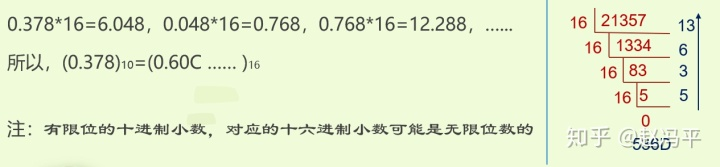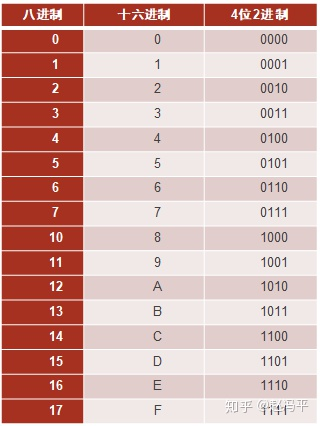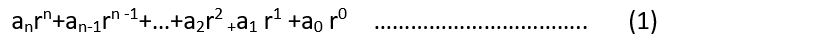# c++十六进制转十进制_十六进制数、八进制数

### 十六进制数与十进制数互相转换

0 1 2 3 4 5 6 7 8 9 A B C D E F

16进制数转十进制数

3E03B2.3）=3*16^5+14*16^4+0*16^3+3*16^2+11*16^1+2*16^0+3*16^-1=4064178.18752进制与16进制数互相转换：从2转16进制，从小数点位置开始，向左从低位到高位每4位（最后不足4位在高位补零）二进制数转换为一位16进制数，小数部分向右，每4位（最后不足4位在低位补零）二进制数转换为一位16进制数；反之，从16进制数转2进制数，把每位16进制数字转化为对应的4位（不足4位高位补零）二进制数即可。### 八进制数

8 进制数（673）=6*8^2+7*8^1+3*8^0 =(2^2+2^1)*2^6+(2^2+2^1+2^0)*2^3+(2^1+2^0)*2^0=2^8+2^7 +2^5+2^4+2^3 +2^1+2^0=(110 111 011)### r进制数就是表示：r进制数的四则运算与十进制的规则一样，r进制数中运算时进退位的法则是“逢r进一，退一当r”。

(1)式在十进制体系下运算的结果，就是r进制数转换成十进制数的值。
11-244812-21369
11-2426
12-03215
12-282829
08-29346
08-142628
02-23113
12-2484
11-011071
01-0852
04-211050
02-11899
11-2840
©️2020 CSDN 皮肤主题: 数字20 设计师:CSDN官方博客